### Vol 17, No 10 (2017) / Wei

Quark stars with strong magnetic fields: considering different magnetic field geometries

# Quark stars with strong magnetic fields: considering different magnetic field geometries

Wei Wei1, , Liu Xi-Wei1, Zheng Xiao-Ping2

College of Science, Huazhong Agricultural University, Wuhan 430070, China
College of Physical Science and Technology, Central China Normal University, Wuhan 430079, China

† Corresponding author. E-mail: weiwei1981@mail.hzau.edu.cn

Abstract: Abstract

We calculate the mass-radius relationship of quark stars with the magnetized density-dependent quark mass model in this work, considering two magnetic field geometries: a statistically isotropic, tangled field and a force-free configuration. In both cases, magnetic field production decreases in the case of maximum quark star mass. Furthermore, a tangled, isotropic magnetic field has a relatively smaller impact on the mass and radius, compared to the force-free configuration, which implies that the geometry of the interior magnetic field is at least as important as the field strength itself when the influence of the strong magnetic field on the mass and radius is assessed.

Keywords: stars: neutron;equation of state;magnetic fields

## 1 Introduction

A neutron star, one of the densest objects in the universe, is an important probe for theories of extreme physics. Since the density in the interior of neutron stars is up to the ground state density of atomic nuclei, neutron stars are probably composed of pure quark matter or quark matter enveloped by nuclear crusts, called quark stars. Different quark matter models can be tested by calculating the structure of quark stars and comparing them with observations. In particular, observations of neutron stars with very high masses are useful. If each set of models predicts a maximum mass lower than the observations, which models should be excluded? For example, observations of a 1.97 ± 0.04 M pulsar and a 2.01 ± 0.04 M pulsar (Demorest et al. ; Antoniadis et al. ) have been used to significantly constrain viable quark star models.

Quark stars are not only extremely dense, but also associated with strong magnetic fields, where the estimated surface value is typically ~ 1012 − 1013 G. In addition, a few rotating radio transients and X-ray dim isolated neutron stars have been observed with even higher magnetic fields (Popov et al. ). Furthermore, soft gamma-ray repeaters and anomalous X-ray pulsars have been reported with the highest magnetic field in neutron stars by using a unified model of magnetars to explain their observed features (Duncan & Thompson ; Paczynski ; Thompson & Duncan ; Melatos ). Various observations of magnetars indicate a surface magnetic field value of ~ 1015 G, and a catalog of 26 known magnetars has been presented recently (Olausen & Kaspi ).

As quark stars with strong magnetic fields appear to exist in nature, many works have been done on the properties of magnetized strange quark matter (MSQM) with various phenomenological confinement models. The significant effect of a strong magnetic field on the equation of state (EOS) of quark matter, described by the conventional MIT bag model, was found by Chakrabarty (). The quasiparticle model, in which the effective quark mass varies with environment, was successfully used by many researchers to probe dense MSQM (Wen et al. ). The special properties of MSQM as described by the Nambu-Jona-Lasinio model have also been investigated (Frolov et al. ; Fayazbakhsh & Sadooghi ).

Another alternative description of strange quarkmatter (SQM) is called the density-dependent quark mass model (DDQM), in which quark mass is dependent on baryon density that is treated as a description of the confinement of the SQM (Plümer et al. ). The DDQM is stiffer than the other models, because the variation of quark mass with density is effectively equivalent to the inclusion of first-order QCD coupling correction along with confinement. Quark stars described by the DDQM with strong magnetic fields were studied by Anand et al. ().

However, one is tempted to ask how the magnetic field geometry affects the structure of quark stars, in addition to magnetic field strength. The purpose of this work is to probe the effect of magnetic field geometry on the mass-radius distribution of quark stars, by considering two different field configurations: a statistically isotropic, tangled field and a force-free configuration (Kamiab et al. ).

The paper is arranged as follows. Section 2 is devoted to the EOS of the MQSM. The mass-radius (MR) relations with an isotropic magnetic field and a forcefree one are described in Sections 3 and 4, respectively. Section 5 presents our conclusions.

## 2 Equation of state of magnetized strange quark matter

Here the DDQM model calculated by Anand et al. () is employed to describe the EOS of MSQM. We treat SQM as a free Fermi gas of u, d, s quarks and electrons, in which the mass of each quark is parameterized as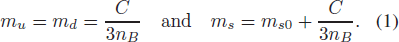Here C is a constant, n B is the baryon number density of the quark matter and m s0 is the strange quark mass. The ranges of these parameters are constrained by the stability conditions (Benvenuto & Lugones ; Wei & Zheng ).

Considering the u, d, s, e system in the presence of a magnetic field B directed along the z-axis, the energy of a charged particle is given by (Landau & Lifshitz )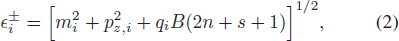where +(−) refers to spin-up (-down) states of the particle, mi and qi are mass and charge of the particle respectively, and pz is the momentum along the z-axis. The thermodynamic potential of the particle is as follows.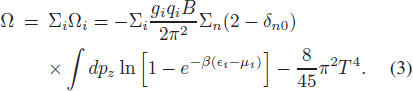The last term is the contribution of gluons, i = (u, d, s, e), and g = 6 for (u, d, s) and 2 for an electron. The pressure Pi , energy density ϵi and baryon number density ni are obtained: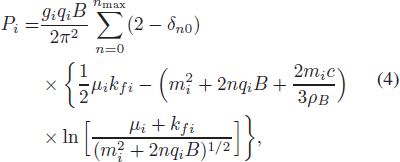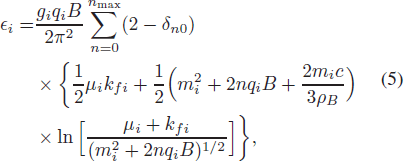and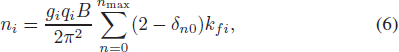where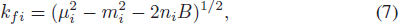and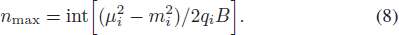The values C = 75 MeV fm−3 and m s0 = 140 MeV are used in the following calculations. The pressure and energy density are now generally functions of the matter density and magnetic field strength.

The primary process responsible for amplification of the magnetic field is believed to be dynamos driven by differential rotation and convection, which produce a tangled magnetic field inside the neutron star Duncan & Thompson ; Thompson & Duncan ). Various studies suggest that these dynamos naturally saturate at a locally equipartition-strength magnetic field during the initial formation of the proto-magnetar after the supernova explosion, while different layers of the remaining stellar core condense to form the neutron star (Chevalier ; Naso et al. ). Making a handful of simplified assumptions and regarding the outcome of the dynamo process (Kamiab et al. ), we expect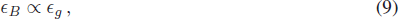where ϵg and ϵB are the local energy density of the gas and the magnetic field, respectively. Since Pg ϵg /3 at this time, this immediately implies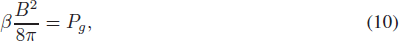where β is the standard proportionality factor relating the gas and the magnetic pressure.

This relation naturally provides a profile of the magnetic field that is proportional to the pressure of the matter. Given a global value of β, we may identify a unique magnetic pressure at each density. For simplicity, we assume spherical symmetry throughout this work and β remains fixed during the proto-magnetar’s formation, following the quenching of the dynamos. Equations (1)–(10) enable us to compute the pressure, energy density and baryon number density with consideration of the beta equilibrium and electric charge neutrality. Examples of the EOS with different values of β are shown in Figure 1.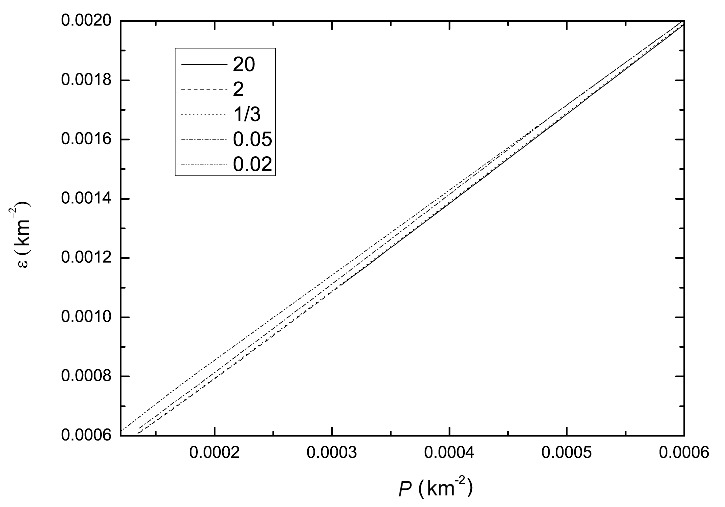Fig. 1 Pressure (P) versus energy density (ϵ) for different values of the magnetic field strength (B) with different values of β. Smaller values of β are associated with higher values of the magnetic field strength.

## 3 M-R relations with an isotropic magnetic field

Choosing a unique EOS of the MSQM (as seen in Fig. 1), and assuming spherical symmetry and isotropy, the M-R relations are obtained by solving the Tolman-Oppenheimer-Volkoff equations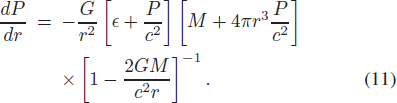In addition to modifying the EOS of the quark matter, the strong interior magnetic field may produce magnetic stress directly. Assuming that the outcome of the dynamo process is a small-scale, tangled field, which is weakly uncorrelated with the generating currents, the resulting stress may be completely described by a magnetic pressure, which is given by PB = (1/3)(B 2/8π). The energy density of the magnetic field is given by ϵB = B 2/8π, where B is fixed by the choice of β. Smaller values of β are associated with higher values of magnetic field strength in quark stars.

The total pressure and energy density are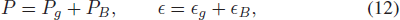where Pg and ϵg are pressure and energy density of the gas respectively. Here the magnetic field provides hydrostatic support for the star by entering globally in the Einstein equations.

Figure 2 shows the M-R relations with different magnetic field strengths by using different values of β. The mass and radius of the quark stars decrease with the effect of the magnetic fields, and larger magnetic field strengths are associated with smaller maximum mass and radius in quark stars. According to Equation (10), β = 1/3 corresponds to a quark star with equal pressure in the magnetic field and matter. In this condition, we get a maximum mass 6.6 percent smaller than the one without the magnetic field.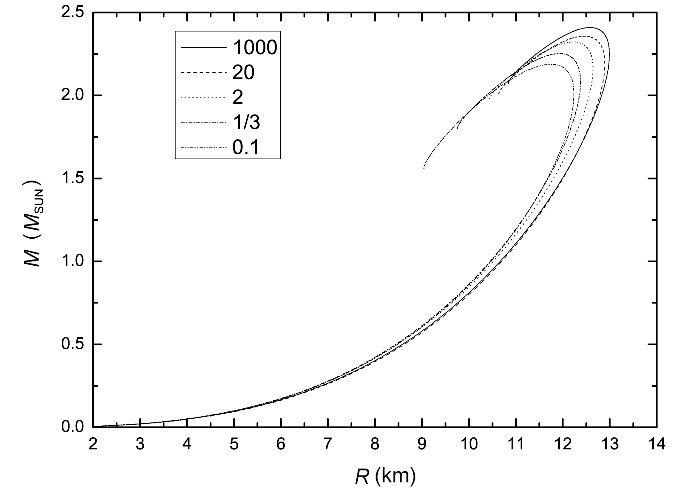Fig. 2 The mass-radius relation for the magnetized EOS with an isotropic magnetic field. The calculation has been done for different values of β in Eq. (10).

Figure 3 displays the magnetic field strengths in the center of different mass quark stars with different values of β. The central magnetic field strength is around 1013 G, when the quark star’s mass reaches its maximum, which is reasonable according to our understanding of the magnetic fields of neutron stars.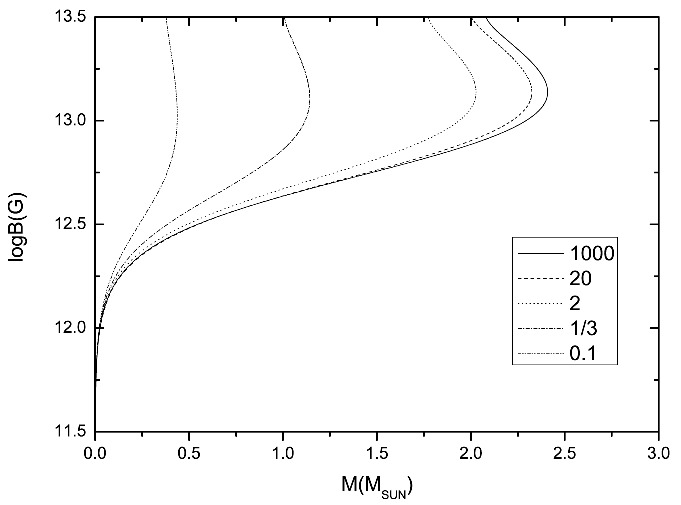Fig. 3 The central magnetic field strengths in different mass quark stars with different β values.

To illustrate the effect of magnetic field strength on the maximum mass and radius of the quark star, Figures 4 and 5 show the maximum mass and radius as functions of 1/β, respectively. These relative changes are fit well by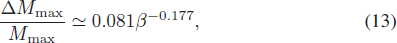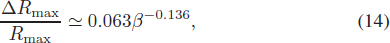for an isotropic tangled magnetic field distribution.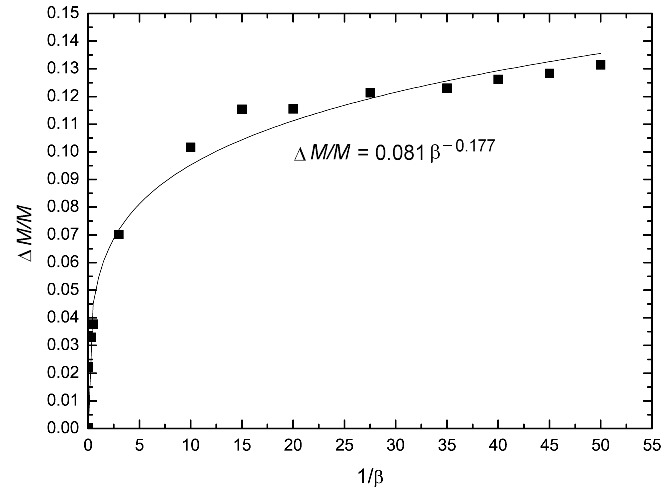Fig. 4 Change in maximum mass of the quark star as a function of 1/β for the mass-radius relation shown in Fig. 2.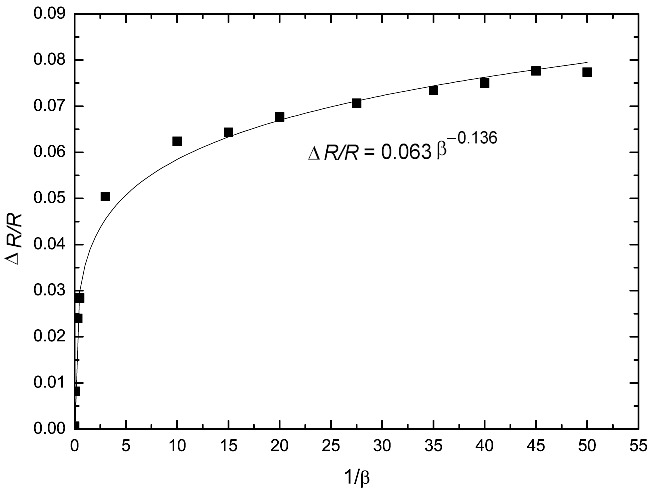Fig. 5 Change in radius of the quark star as a function of 1/β for the mass-radius relation shown in Fig. 2.

## 4 M-R relations with a force-free magnetic field

Despite the small-scale, turbulent field that is believed to be initially produced by the dynamos, the final magnetic field configuration remains unclear. In this paper, regarding the magnetic field geometry: a force free configuration is also considered. After dynamo quenching and before the formation of a crust, the quark star will reconfigure the magnetic field geometry via bulk fluid motions, resulting in a linked, nearly force-free geometry that is decided by the initial magnetic helicity (Braithwaite & Spruit , ). This is a natural consequence of helicity conservation, corresponding to the minimum energy state at fixed magnetic helicity (Broderick & Narayan ). To assess the effect of the force-free condition on hydrostatic equilibrium of the star, we need to solve the Newton-Euler equation and impose static conditions. According to the results of Kamiab et al. (), in dimensions where G = 1 and c = 1, the Newton-Euler equation coming from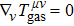is given by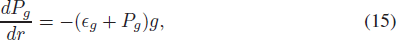where g is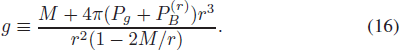Using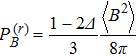and the equipartition assumption, the total radial pressure becomes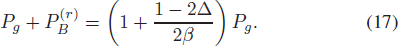Δ is an anisotropy parameter that quantifies the anisotropy of this average configuration. An anisotropy parameter Δ = −1 corresponds to a purely tangential magnetic field configuration and Δ = 2 corresponds to a purely radial field. An isotropic average field will have Δ = 0. We refer to the isotropic core with Δ = 0 in a range of radii from 0 to 3 km.

Using the EOS of the DDQM model (Fig. 1) and Equations (15)–(17), the resulting M-R relationships from numerical integration are shown in Figure 6, in which results based on an isotropic magnetic field are also plotted for comparison.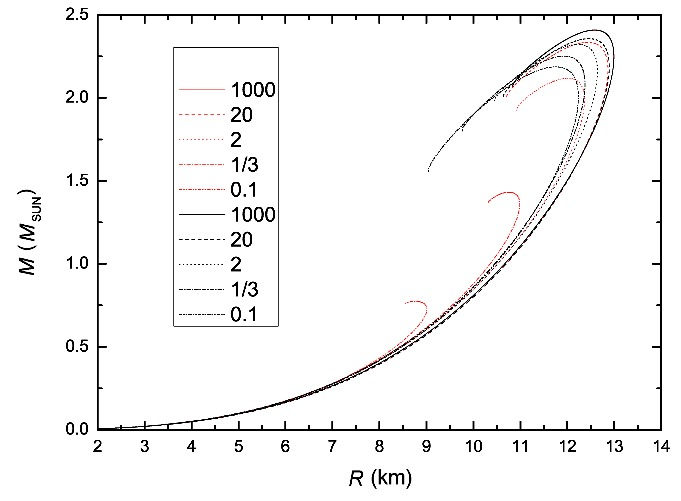Fig. 6 The M-R relation for the MSQM. The red curves are for a force-free configuration of the magnetic field and the black curves correspond to an isotropic one.

In contrast to the case of a tangled, isotropic magnetic field, the force-free configuration produces a more obvious decrease in the maximum mass with the same value of β. The smaller the β is, the stronger the magnetic field is, and the larger the difference in the maximum mass between the two configurations is.

Figure 7 explicates the central magnetic field strengths of the quark stars with a force-free configuration. The central magnetic field strength is around 1013 G when the quark star’s mass reaches its maximum, which is similar to the isotropic case. As before, changes in mass and radius are fit well by the power law functions,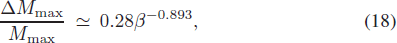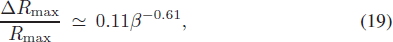which are illustrated in Figures 8 and 9.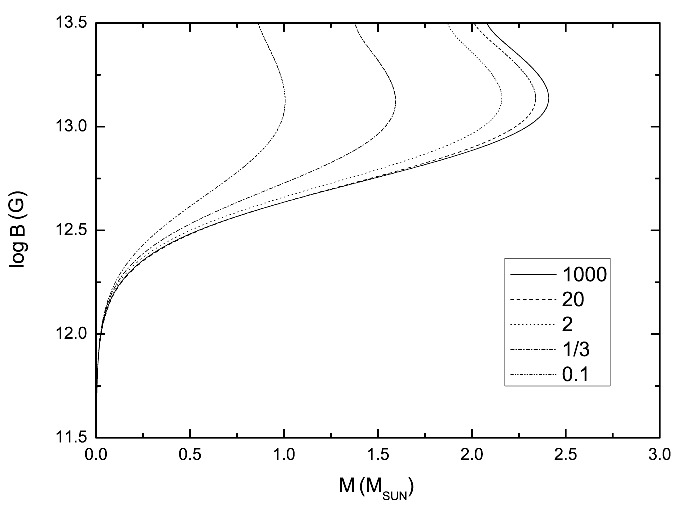Fig. 7 The central magnetic field strengths in different mass quark stars with different β values.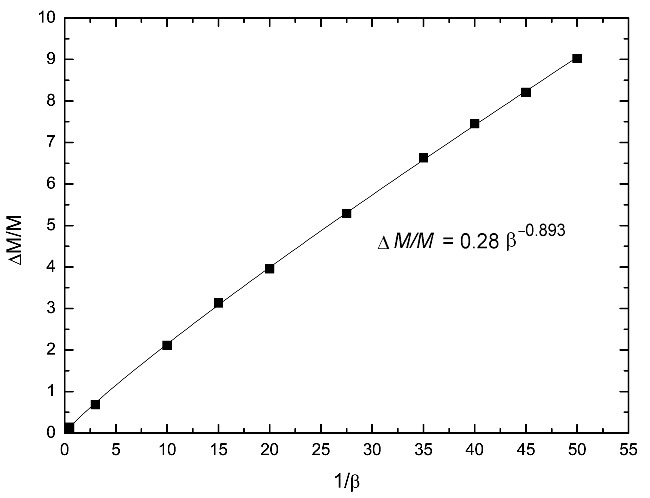Fig. 8 Change in maximum mass of the quark star as a function of 1/β for the force-free configuration of the magnetic field.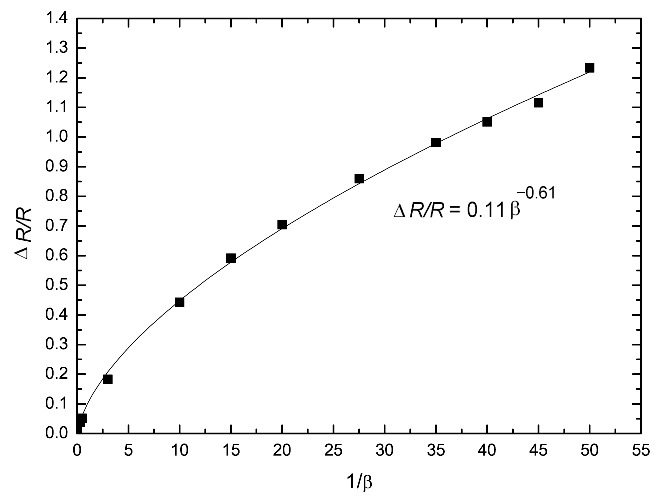Fig. 9 Change in radius of the quark star as a function of 1/β for the force-free configuration of the magnetic field.

## 5 Conclusions

In this paper, we have shown that the assumed geometry of the magnetic field in a quark star is at least as important as the field strength itself, when the impact of the magnetic field on mass and radius is assessed. Both the tangled, isotropic magnetic configuration and force-free one produce decreases in the maximum quark star mass. Furthermore, a tangled, isotropic magnetic field has a smaller impact on the mass, compared to the force-free configuration.

Although the magnetic configurations obviously reduce the maximum mass, calculations in this study indicate that quark stars described by the DDQM model are compatible with observations of massive neutron stars with mass ≥ 2 M . Nevertheless, these results challenge the assertion that magnetic fields provide a means to reconcile the recent observations of very massive neutron stars with relatively soft EOS of the quark matter, whose calculated maximum mass is otherwise precluded. Some EOSs of quark matter, such as the MIT bag model and quark quasi-particle model, support values of maximum mass that are lower than 1.6 M . Although many works found that the effect of strong interactions, such as one-gluon exchange or color-superconductivity, can stiffen the EOS of quark matter, strong magnetic fields change the structure of the quark stars and reduce the mass, which introduce difficulties in explaining the astronomical observations of massive neutron stars.

An interesting possibility is that the relation between the change of mass and internal magnetic field strength (Figs. 45, 89) may provide a new way to distinguish the internal magnetic field configuration (isotropic vs. force free) or to probe the dense matter (nucleon vs. quark) in neutron stars. Different neutron stars might have dynamos with varying efficiency during their formation processes. Future observations of the M-R relationship of neutron stars may exhibit a more intrinsic scatter related to their internal magnetic field. Now the magnetic field in the interior of neutron stars cannot be directly measured, but it is generally estimated by using the virial theorem. If the internal magnetic field can be measured in the future, which could correlate with this scatter and provide a sign of correlation, it would be indicative of the field configuration and dense matter in neutron stars.

 Anand J. D. Chandrika Devi N. Gupta V. K. Singh S. 2000 ApJ 538 870 Antoniadis J. Freire P. C. C. Wex N. et al. 2013 Science 340 448 Benvenuto O. G. Lugones G. 1998 International Journal of Modern Physics D 7 29 Braithwaite J. Spruit H. C. 2004 Nature 431 819 Braithwaite J. Spruit H. C. 2006 A&A 450 1097 Broderick A. E. Narayan R. 2008 MNRAS 383 943 Chakrabarty S. 1996 Phys. Rev. D 54 1306 Chevalier R. A. 2005 ApJ 619 839 Demorest P. B. Pennucci T. Ransom S. M. Roberts M. S. E. Hessels J. W. T. 2010 Nature 467 1081 Duncan R. C. Thompson C. 1992 ApJ 392 L9 Fayazbakhsh S. Sadooghi N. 2011 Phys. Rev. D 83 025026 Frolov I. E. Zhukovsky V. C. Klimenko K. G. 2010 Phys. Rev. D 82 076002 Kamiab F. Broderick A. E. Afshordi N. 2015 arXiv: 1503.03898 Landau L. D. Lifshitz E.M. 1980 Statistical Physics. 1 2 Oxford Pergamon Press Melatos A. 1999 ApJ 519 L77 Naso L. Rezzolla L. Bonanno A. Paternò L. 2008 A&A 479 167 Olausen S. A. Kaspi V. M. 2014 ApJS 212 6 Paczynski B. 1992 Acta Astronomica 42 145 Plümer M. Raha S. Weiner R. M. 1984 Physics Letters B 139 198 Popov S. B. Turolla R. Possenti A. 2006 MNRAS 369 L23 Thompson C. Duncan R. C. 1993 ApJ 408 194 Thompson C. Duncan R. C. 1995 MNRAS 275 255 Wei W. Zheng X.-P. 2012 Astroparticle Physics 37 1 Wen X.-J. Su S.-Z. Yang D.-H. Peng G.-X. 2012 Phys. Rev. D 86 034006
Cite this article: Wei Wei, Liu Xi-Wei, Zheng Xiao-Ping. Quark stars with strong magnetic fields: considering different magnetic field geometries. Cancer Biol Med. 2017; 10:102.

### Refbacks

• There are currently no refbacks.